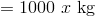Archimedes’ Principle and Buoyancy

If an object is submerged in a liquid, the object will displace a volume of the liquid equal to the volume of the submerged object.  Legend has it that this observation was made by Archimedes when he sat in a bath tub that was filled to the top of the tub.  The volume of water that overflowed was equal to the volume of the bather.  The forces exerted by the fluid on the sides of the submerged object are balanced but the forces exerted by the fluid on the top and bottom of the object are not equal.  The force exerted on the bottom of the object is greater than the force exerted by the liquid on the top.  Therefore, the liquid exerts a net upward force on the submerged or floating object.  The force is called buoyancy and its magnitude is equal to the weight of the displaced water.  Archimedes Principle states that the buoyant force is equal to the weight of the displaced liquid.

Example Problem: The density of steel is 9000. kg/m3 and the density of water is 1000. kg/m3.  If a cube of steel that is 0.100 m on each side is placed in a tank of water and weighed while under water, what is the apparent weight of the cube?

Solution: The volume of the cube is 0.00100 m3.

The mass of the cube is 9.00 kg.

The weight of the cube when not submerged in water = (9.00 kg)(9.80 m/s2) = 88.2 N

The mass of water displaced by the cube = 1.00 kg

The weight of the water displaced by the cube = 9.80 N

The buoyant force on the steel cube = 9.80 N

Apparent weight of cube under water = 88.2 N - 9.80 N = 78.4 N

Example Problem: A hollow metal cube 1.00 m on each side has a mass of  600. kg.  How deep with this cube sink when placed in a vat of water?

Solution: Since the weight of the cube is 5880 N, it will need to displace 5880 N of water in order to float.

Volume of submerged portion of cubeMass of water displacedWeight of water displacedThe cube will sink to the level that 0.60 meters are under water and 0.40 meters are above water.

#### Summary

• If an object is submerged in a liquid, the object will displace a volume of the liquid equal to the volume of the submerged object.
• The forces exerted by the fluid on the sides of the submerged object are balanced but the forces exerted by the fluid on the top and bottom of the object are not equal.
• The liquid exerts a net upward force on the submerged or floating object.
• This force is called buoyancy and its magnitude is equal to the weight of the displaced water.
• Archimedes Principle states that the buoyant force is equal to the weight of the displaced liquid.